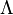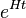Lets look at an early universe model made entirely of classical General Relativity. Multiply connected, very lumpy, with energy across huge bandwidths.

Lots of energy – some 10^80 nucleons worth, all in some region with small finite volume. How would this smooth itself out as time evolves?

Are fundamental particles at their core an echo of the conditions at the big bang? In other words the density of energy in g/cm^3 of the core of an electron is perhaps the same energy density at which electrons were formed.

#### Crazy thought:

I think that electrons are much much smaller than quarks, and as such formed earlier in the big bang.  This was the start of inflation. The universe consisted of electrons + other chaotic GR mess. So we have incredible expansion as the electrons repel each other ferociously.

Then as time passed, and the universe approached the meter size, quarks and nucleons organized to quench the repulsion.

According to the standard model of inflation, (see below) that means that electrons are about 10^-77 m across while quarks are larger, more like 10^-27 meter.  (not sure I did the math right?)

So inflation is a phenomenon of the creation of charge in the Universe.

Reading a little on this – its at odds with the current theory (no doubt !) – in that the current theory has inflation coming when the strong nuclear force is separating out. But perhaps that’s another way to look at it – there are no forces other than random chaotic ones, and electrons give quarks a reason to be created – to soak up the energy of ( or  quench)  the inflation.

Wikipedia

the large potential energy of the inflaton field decays into particles and fills the Universe with Standard Model particles

– electrons and quarks apply brakes to inflation as they condense.

-cosmological constant is bound up spring like effect of noisy GR wave energy piled to the limit of curvature. Once we start to drop density, density drops faster and faster as GR is non linear, so there is less to keep it together. This is the origin of the cosmological constant, which powers inflation:

Wikipedia

This steady-state exponentially expanding spacetime is called a de Sitter space, and to sustain it there must be a cosmological constant, a vacuum energy proportional to$\Lambda$ everywhere. In this case, the equation of state is$\! p=-\rho$. The physical conditions from one moment to the next are stable: the rate of expansion, called the Hubble parameter, is nearly constant, and the scale factor of the Universe is proportional to$e^{Ht}$. Inflation is often called a period of accelerated expansion because the distance between two fixed observers is increasing exponentially (i.e. at an accelerating rate as they move apart), while$\Lambda$ can stay approximately constant (see deceleration parameter).

The basic process of inflation consists of three steps:
1. Prior to the expansion period, the inflaton field was at a higher-energy state.
2. Random quantum fluctuations triggered a phase transition whereby the inflaton field released its potential energy as matter and radiation as it settled to its lowest-energy state.
3. This action generated a repulsive force that drove the portion of the Universe that is observable to us today to expand from approximately 10−50 metres in radius at 10−35 seconds to almost 1 metre in radius at 10−34 seconds.